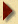All the doors which correspond to a square number will be open, the rest will be shut.
Why? well the doors are only visited by the students whose number can divide them, for instance door 6 is visited by #1, #2, #3 and #6, since there is an even number of visitors to this door, it will be shut as a pair of visitors cause and open/shut pair set.
All non square numbers have an even number of divisors, for instance 6 is:

6 = 1x6
6 = 2x3
6 = 3x2
6 = 6x1
They go by pair because a high number always mates with a low number to yield the result. In the case of squares, there is an additional set, in the middle which is unique because it is its own matching pair so 36 for instance is:
36 = 1x36
36 = 2x18
36 = 3x12
36 = 4x9
36 = 6x6 <-- This one is unique Sqrt x Sqrt
36 = 9x4
36 = 12x3
36 = 18x2
36 = 36x1
Hence door 36 will be visited "9" times, 8 times by pairs of students like pair 2 and 18, and once by student "6 = sqrt(36)" which will cause the door to remain open at the end
The doors 1, 4, 9, 16, 25, 36, 49, 64, 81, 100 will be open, all other doors will be shut.Puzzle Page